Thursday, August 5, 2021
Home > Common Core Algebra 1 > Common Core Algebra 1 Unit 4 Functions Chapters List

# Common Core Algebra 1 Unit 4 Functions Chapters List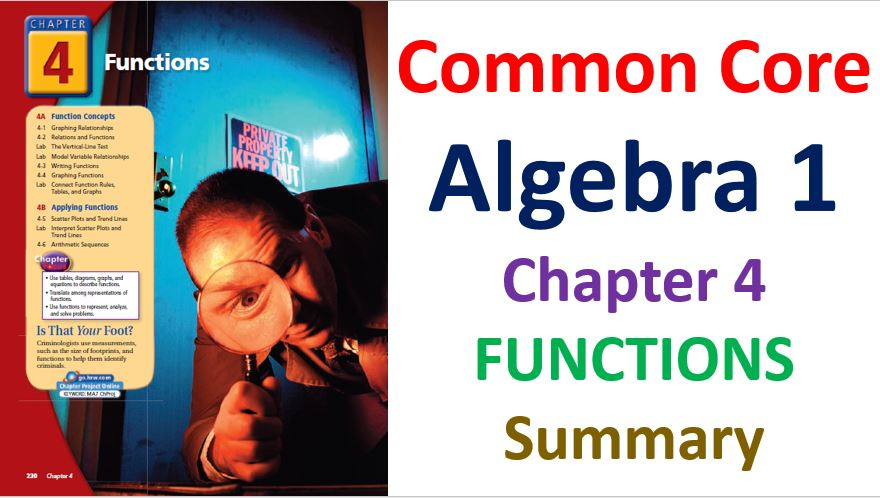# Common Core Algebra 1 Unit 4 Functions Chapters List

Hi Students, Welcome to Amans Maths Blogs (AMB). In this article, you get the full details of Common Core Algebra 1 Unit 4 Functions Chapters List and Summary. It means, I provide you short details of chapters of Common Core Algebra 1 Unit 4 Functions Chapters. Some definitions and important questions with answers and solutions.

## Common Core Algebra 1 Unit 4A Functions Concepts

Now, there are following chapters in Common Core Algebra 1 Unit 4A Functions Concepts

### Common Core Algebra 1 Unit 4A Chapter 1 Graphing Relationships

In this chapter, you will learn about how to graph a relationship.

Graphs can be used to illustrate many different situations.

Some graphs are connected lines or curves called continuous graphs.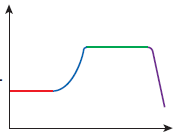Some graphs are only distinct points. These are called discrete graphs. In this case, the values between two dots are not included as they have no meaning in the given situation.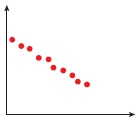Lets understand it by solving some questions:

Common Core Algebra 1 Unit 4A Chapter 1 Question : 1

Fill in the blank: A ______ graph is made of connected lines or curves. (continuous, discrete)

Common Core Algebra 1 Unit 4A Chapter 1 Solution : 1

connected  graph is made of connected lines or curves.

Common Core Algebra 1 Unit 4A Chapter 1 Question : 2

Fill in the blank: A ______ graph is made of distinct points. (continuous, discrete)

Common Core Algebra 1 Unit 4A Chapter 1 Solution : 2

discrete  graph is made of distinct points.

### Common Core Algebra 1 Unit 4A Chapter 2 Relations and Functions

In this chapter, you will learn about what the relation and function are and how to find the domain and range of the relation and functions.

In previous chapter, you see that a relationship is represented by a graph. The relationships can also be represented by a set of order pairs, called a relation.

A relation can also be represented by tables, graphs or mapping diagrams.

Example: The relation {(1, 5), (2, 3), (3, 2), (4, 1)} can be represented as a table, as a graph and as a mapping diagram.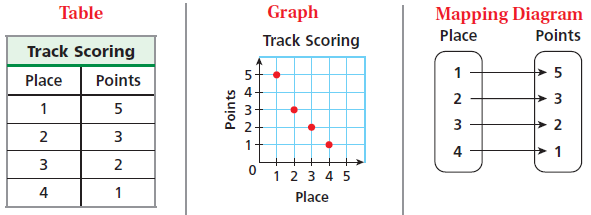The domain of a relation is the set of first coordinate (x-coordinate) of the ordered pairs and the range of a relation is the set of second coordinate (y-coordinate) of the order pairs.

For example: In the relation {(1, 5), (2, 3), (3, 2), (4, 1)}, the domain is {1, 2, 3, 4} and the range is {5, 3, 2, 1}.

A function is a special type of relation that pairs each domain value with exactly one range value.

Lets understand it by solving some questions:

Common Core Algebra 1 Unit 4A Chapter 2 Question : 1

What is the domain and range of {(-5, 7), (0, 0), (2, -8), (5, -20)}

Common Core Algebra 1 Unit 4A Chapter 2 Solution : 1

Domain of given relation is {-5, 0, 2, 5} and Range of given relation is {7, 0, -8, -20}.

Common Core Algebra 1 Unit 4A Chapter 2 Question : 2

What is the domain and range of {(1, 2), (2, 4), (3, 6), (4, 8), (5, 10)}.

Common Core Algebra 1 Unit 4A Chapter 2 Solution : 2

Domain of given relation is {1, 2, 3, 4, 5} and Range of given relation is {2, 4, 6, 8, 10}.

Common Core Algebra 1 Unit 4A Chapter 2 Question : 3

What is the domain and range of the relation as given in table form below.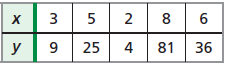Common Core Algebra 1 Unit 4A Chapter 2 Solution : 3

Domain of given relation is {3, 5, 2, 8, 6} and Range of given relation is {9, 25, 4, 81, 36}.

### Common Core Algebra 1 Unit 4A Chapter 3 Writing Functions

In this chapter, you will learn about how to identify independent and dependent variables and how to write an equation in function notation and evaluate a function for given input values.

The input of a function is the independent variable. The output of a function is the dependent variable. The value of the dependent variable depends on the value of independent variables.

Hence, the dependent variable is a function of independent variables. If ‘x’ is the independent variable and ‘y’ is the dependent variable, then the function is written as y = f(x).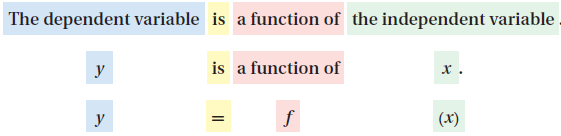Lets understand it by solving some questions:

Common Core Algebra 1 Unit 4A Chapter 3 Question : 1

For the function f(x) = 5x, find value of f(x) at x = 6 and at x = 7.5.

Common Core Algebra 1 Unit 4A Chapter 3 Solution : 1

Given function is f(x) = 5x.

Put x = 6, then f(6) = 5(6) = 30.

Put x = 7.5, then f(7.5) = 5(7.5) = 37.5.

Common Core Algebra 1 Unit 4A Chapter 3 Question : 2

For the function f(x) = 2x – 1, find value of f(x) at x = 1 and at x = -3.

Common Core Algebra 1 Unit 4A Chapter 3 Solution : 2

Given function is f(x) = 2x – 1.

Put x = 1, then f(1) = 2(1) – 1 = 2 – 1 = 1

Put x = -3, then f(-3) = 2(-3) – 1 = -6 – 1 = -7

Common Core Algebra 1 Unit 4A Chapter 3 Question : 3

For the function f(x) = x/4 + 1, find value of f(x) at x = -24 and at x = 400.

Common Core Algebra 1 Unit 4A Chapter 3 Solution : 3

Given function is f(x) = x/4 + 1.

Put x = -24, then f(-24) = -24/4 + 1 = -6 + 1 = -5

Put x = 400, then f(400) = 400/4 + 1 = 100 + 1 = 101

### Common Core Algebra 1 Unit 4A Chapter 4 Graphing Function

In this chapter, you will learn about how to graph the functions for a given limited domain and for all real numbers.

To graph a function for all real numbers, you need to do the following steps:

Step 1: Use the function to generate ordered pairs by choosing several values for x.

Step 2: Plot enough points to see a pattern for the graph.

Step 3: Connect the points with a line or smooth curve.

Lets understand it by solving some questions:

Common Core Algebra 1 Unit 4A Chapter 4 Question : 1

Graph the function f(x) = |x| for the domain {-2, -1, 0, 1, 2}.

Common Core Algebra 1 Unit 4A Chapter 4 Solution : 1

First, you need to find the values of f(x) at x = -2, -1, 0, 1, 2.Now, graph the ordered pair.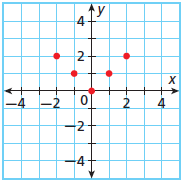Common Core Algebra 1 Unit 4A Chapter 4 Question : 2

Graph the function y = 2x + 1

Common Core Algebra 1 Unit 4A Chapter 4 Solution : 2

First, you need to choose some values of x and find corresponding values of f(x) to generate ordered pairs.Now, plot the points to see a pattern and then connect all the points by a line.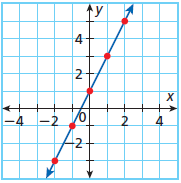## Common Core Algebra 1 Unit 4B Applying Functions

Now, there are following chapters in Common Core Algebra 1 Unit 4B Applying Functions

### Common Core Algebra 1 Unit 4B Chapter 5 Scatter Plots and Trends Line

In this chapter, you will learn about how to create and interpret scatter plots and how to use trend lines to make prediction.

A scatter plot  is a graph with points plotted to show a possible relationship between two sets of data. A scatter plot is an efficient way to display some types of data.

A correlation describes a relationship between two data sets. A graph may show the correlation between data. The correlation can help you analyze trends and make the prediction.

There are three types of correlation between the data: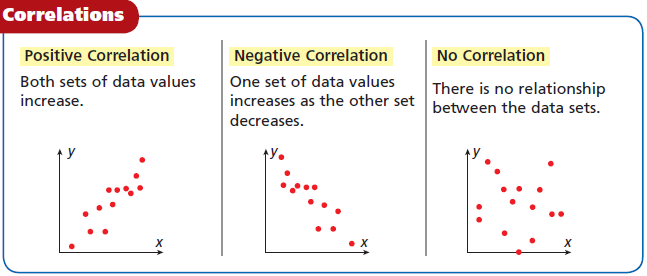Lets understand it by solving some questions:

Common Core Algebra 1 Unit 4B Chapter 5 Question : 1

The table shows the number of species added to the list of endangered and threatened species in the UNITED STATES during the given years. Graph a scatter plot using the given data.Common Core Algebra 1 Unit 4B Chapter 5 Solution : 1

Required scatter plot of the given table data is below.### Common Core Algebra 1 Unit 4B Chapter 6 Arithmetic Sequence

In this chapter, you will learn about arithmetic sequence and how to find its nth term.

A sequence is a list of numbers that may form a pattern. Each number in a sequence is term.

When the terms of a sequence differ by the same nonzero number d, the sequence is an arithmetic sequence and d is the common difference.

To find a term in an sequence, you need to add d to its previous term. The formula to find nth term of arithmetic sequence isets understand it by solving some questions:

Common Core Algebra 1 Unit 4B Chapter 6 Question : 1

Determine whether the sequence appears to be an arithmetic sequence. If so, find the common difference: 12, 8, 4, 0, ….

Common Core Algebra 1 Unit 4B Chapter 6 Solution : 1

Given sequence is 12, 8, 4, 0, ….

Since

8 – 12 = -4

4 – 8 = -4

0 – 4 = -4… it means this is in arithmetic sequence and its common difference is -4

Common Core Algebra 1 Unit 4B Chapter 6 Question : 2

Find 60th term of the arithmetic sequence : 11, 5, -1, -7, …

Common Core Algebra 1 Unit 4B Chapter 6 Solution : 2

Given arithmetic sequence : 11, 5, -1, -7, …

We have a1 = 11, d = 5 – 11 = -6 and n = 60.

Using formula an = a1 + (n – 1)d, we get

a60 = 11 + (60 – 1)(-6) = 11 – 6 x 59 = – 343

Common Core Algebra 1 Unit 4B Chapter 6 Question : 3

Find the common difference for the arithmetic sequence 107, 105, 103, 101, …

Common Core Algebra 1 Unit 4B Chapter 6 Solution : 3

Given arithmetic sequence : 107, 105, 103, 101, …

Required difference is d = 105 – 107 = -2

Common Core Algebra 1 Unit 4B Chapter 6 Question : 4

Find 17th term of the arithmetic sequence : 5, 10, 15, 20, 25, …

Common Core Algebra 1 Unit 4B Chapter 6 Solution : 4

Given arithmetic sequence : 5, 10, 15, 20, 25, …

We have a1 = 5, d = 10 – 5 = 5 and n = 17.

Using formula an = a1 + (n – 1)d, we get

a17 = 5 + (17 – 1)(5) = 5 + 80 = 85

error: Content is protected !!